# Calculation of Inductance Matrix

In principle, we could determine the relationship between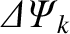and the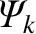(which is equivalent to determining the relationship between the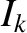and the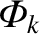) by solving Equations (14.46) and (14.47) subject to suitable spatial boundary conditions at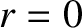and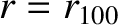[11,14,22]. However, in this chapter, we shall adopt a more direct approach [12,13,15,16].

According to the Biot-Savart law :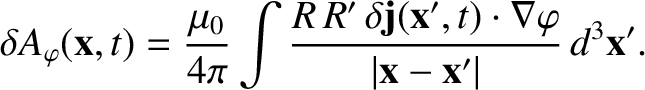(14.102)

Let us assume that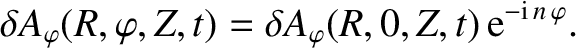(14.103)

It follows that we can evaluate the integral on the right-hand side of Equation (14.102) at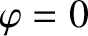without loss of generality. Now,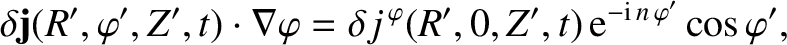(14.104)

so we get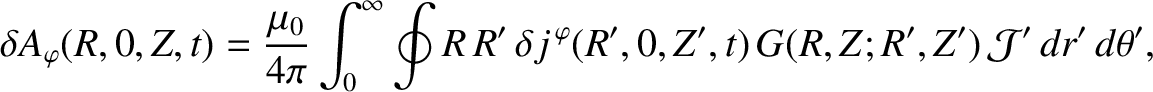(14.105)

where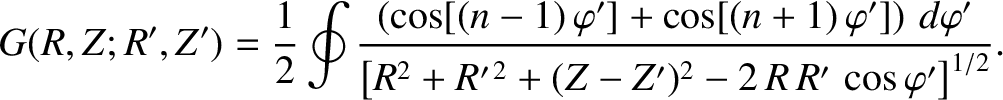(14.106)

Finally, making use of the standard definition of a toroidal function ,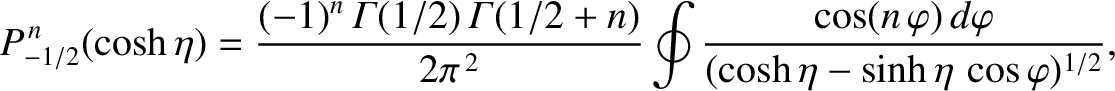(14.107)

where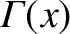denotes a gamma function , we arrive at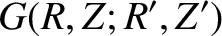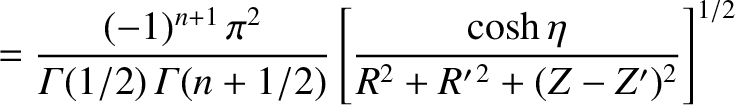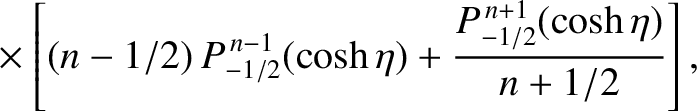(14.108)

where(14.109)

According to Equations (14.60) and (14.66),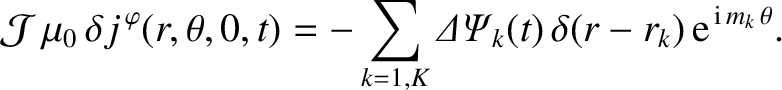(14.110)

Furthermore, Equations (14.65) and (14.78) yield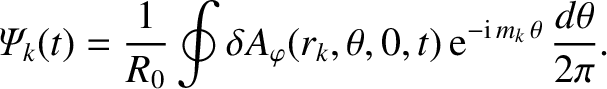(14.111)

Hence, combining the previous two expressions with Equation (14.105), we obtain the expected normalized inductance relation [see Equation (14.94)],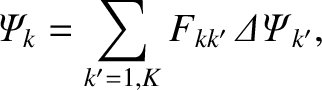(14.112)

for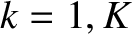, where [12,13,15,16]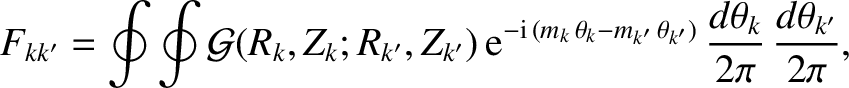(14.113)

and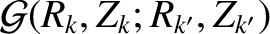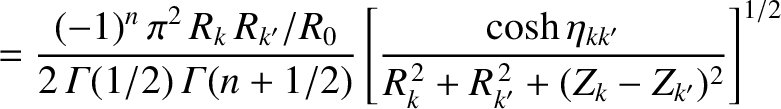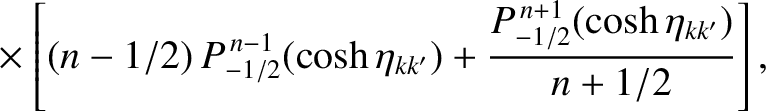(14.114)

with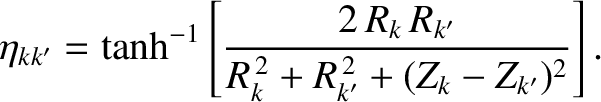(14.115)

Here,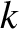and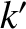index the various rational surfaces in the plasma. Moreover, the double integral in Equation (14.113) is taken around theth rational surface (cylindrical coordinates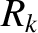, 0,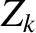; flux coordinates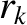,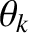, 0, withconstant; resonant poloidal mode number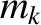) and theth rational surface (cylindrical coordinates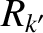, 0,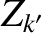; flux coordinates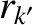,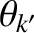, 0, withconstant; resonant poloidal mode number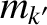).

Note that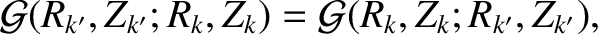(14.116)

which, from Equation (14.113), implies that the F-matrix is Hermitian [see Equation (14.100)], as must be the case.

Finally, according to Equations (14.95) and (14.113), the unnormalized inductance matrix takes the form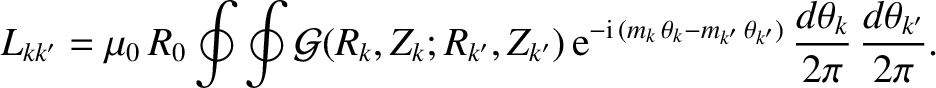(14.117)

The Hermitian L-matrix,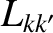, specifies the self and mutual inductances of the helical current sheets that flow at the various rational surfaces within the plasma.

Note that the calculation of the F-matrix outlined in this section is only approximate. The exact calculation is specified in References  and .